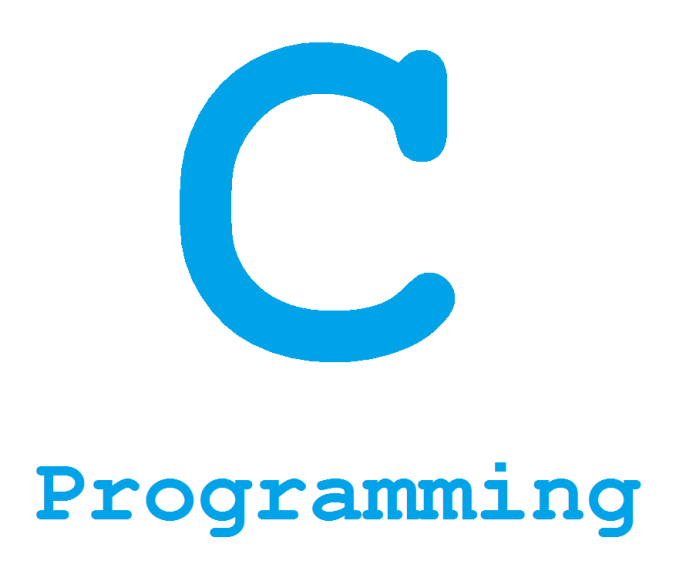こちらは旧ブログになります。

# Recursive functions for people who can not program## Introduction

The school's lesson has followed the C language.

It seems difficult for everyone else's department to learn, so I’ll write a hint.

## How to declare functions

First from the declaration method of the basic function. In order to use the function, prototype declaration is required.

```#include  <stdio.h>
int Rec( int n );   // prototype declaration

int main ( void ) {

// processing
return 0;
}
```

The prototype declaration is easy to manage when many functions come out. The contents of the declared function can be written anywhere afterwards.

```#include  <stdio.h>

int func( int n );   // prototype declaration

int main ( void ) {

// processing

int ans = func( 5 ); // 25

return 0;
}

int func( int n ) {

return n * n;
}
```

Also, you can write it simultaneously with the declaration.

```#include  <stdio.h>

int func( int n ) { // prototype declaration

return n * n;
}

int main ( void ) {

// processing

int ans = func( 5 ); // 25

return 0;
}
```

## Recursive function

• Recursive call is to call yourself “yourself” * You can easily write using a recursive function when describing an algorithm with a recursive structure.

## Factorial

Factorial is a calculation that multiplies from that number to one.

It is the sum from 1 to n.

The following program calculates "n!".

```#include <stdio.h>

int Rec( int n ) {

// processing

// 0! = 1
if ( n == 0 ) {

return 1;
}else {

return n * Rec( n - 1 );
}
}

int main ( void ) {
// abridgement
int ans = Rec( 4 );
printf( "%2d\n", ans ); // 24
}
```

In this case, we shall learn how to call the function

```Rec( 4 ) : 4 * Rec( 3 );
Rec( 3 ) : 3 * Rec( 2 );
Rec( 2 ) : 2 * Rec( 1 );
Rec( 1 ) : 1 * Rec( 0 );
Rec( 0 ) : 1;
```

It becomes like the above. When assigning to each `Rec( 4 ) : 4 * 3 * 2 * 1 * 1 = 24` That means "4! (The factorial of 4)"

## Fibonacci sequence

Next let's compute the Fibonacci sequence The Fibonacci sequence is the sequence of the preceding term and the sequence of two previous terms.

However, in this case, if you want to calculate F50F50 items, you have to calculate all F0F0 to F50F50. Write a general formula for FnFn item below.

The former is suitable for programming. (Unless you care about processing speed) Below is the code for Fibonacci sequence.

```#include <stdio.h>

int fibonacci( int n ) {
switch ( n ) {
case 0: return 0;
case 1: return 1;
default: return fibonacci( n - 2 ) + fibonacci( n - 1 );
}
}

void main(void) {
int n;
printf( "n = " );
scanf( "%d", &n );
printf( "F(%d) = %d\n", n, fibonacci( n ) );
}
```

I think it is a bit difficult to follow the call of this function, but it is easy if you know the idea. When F6F6 is calculated, Fibonacci sequence is`0 1 1 2 3 5 8 13 21 ...` to `F 6 = 8 F 6 = 8`. As the operation of the above program, `n = 6`.

```fibonacci( 6 ) : fibonacci( 4 ) + fibonacci( 5 )
fibonacci( 5 ) : fibonacci( 3 ) + fibonacci( 4 )
fibonacci( 4 ) : fibonacci( 2 ) + fibonacci( 3 )
fibonacci( 3 ) : fibonacci( 1 ) + fibonacci( 2 )
fibonacci( 2 ) : fibonacci( 0 ) + fibonacci( 1 )
fibonacci( 1 ) : 1
fibonacci( 0 ) : 0

// When assigning each

fibonacci( 6 ) : fibonacci( 4 ) + fibonacci( 5 ) // 8
fibonacci( 5 ) : fibonacci( 3 ) + fibonacci( 4 ) // 5
fibonacci( 4 ) : fibonacci( 2 ) + fibonacci( 3 ) // 3
fibonacci( 3 ) : fibonacci( 1 ) + fibonacci( 2 ) // 2
fibonacci( 2 ) : fibonacci( 0 ) + fibonacci( 1 ) // 1
```

And I was able to find `F6 = 8 F6 = 8`.

## Conclusion

It is much easier to organize by self-calling without composing with or for something when constructing programs that repeat similar things like this.